Test: Transistor Biasing & Thermal Stabilization- 2

# Test: Transistor Biasing & Thermal Stabilization- 2

Test Description

## 15 Questions MCQ Test Topicwise Question Bank for Electrical Engineering | Test: Transistor Biasing & Thermal Stabilization- 2

Test: Transistor Biasing & Thermal Stabilization- 2 for Electrical Engineering (EE) 2023 is part of Topicwise Question Bank for Electrical Engineering preparation. The Test: Transistor Biasing & Thermal Stabilization- 2 questions and answers have been prepared according to the Electrical Engineering (EE) exam syllabus.The Test: Transistor Biasing & Thermal Stabilization- 2 MCQs are made for Electrical Engineering (EE) 2023 Exam. Find important definitions, questions, notes, meanings, examples, exercises, MCQs and online tests for Test: Transistor Biasing & Thermal Stabilization- 2 below.
Solutions of Test: Transistor Biasing & Thermal Stabilization- 2 questions in English are available as part of our Topicwise Question Bank for Electrical Engineering for Electrical Engineering (EE) & Test: Transistor Biasing & Thermal Stabilization- 2 solutions in Hindi for Topicwise Question Bank for Electrical Engineering course. Download more important topics, notes, lectures and mock test series for Electrical Engineering (EE) Exam by signing up for free. Attempt Test: Transistor Biasing & Thermal Stabilization- 2 | 15 questions in 45 minutes | Mock test for Electrical Engineering (EE) preparation | Free important questions MCQ to study Topicwise Question Bank for Electrical Engineering for Electrical Engineering (EE) Exam | Download free PDF with solutions
 1 Crore+ students have signed up on EduRev. Have you?
Test: Transistor Biasing & Thermal Stabilization- 2 - Question 1

### Assertion (A): Current mirrors are widely used in analog integrated circuits for DC biasing. Reason (R): Current mirrors provide highly stable DC bias points without the need of a lot of biasing resistors.

Test: Transistor Biasing & Thermal Stabilization- 2 - Question 2

### Consider the following statements: 1. Self-blas is superior to the fixed bias from the consideration of stability of operating point in the CE amplifier. 2. Bias stabilization is used to prevent thermal runaway. 3. Improper biasing of a transistor results in the distortion of the output signal. 4. A transistor is said to be in quiescent stage when no signal is applied to the input. Q. Which of the statements given above are correct?

Test: Transistor Biasing & Thermal Stabilization- 2 - Question 3

### For the circuit shown below, what is the value of RC needed if VC = 2 V ?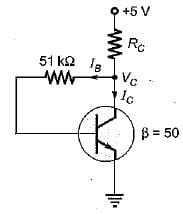Detailed Solution for Test: Transistor Biasing & Thermal Stabilization- 2 - Question 3

Using KVL, we have:
-5 + RC (IB + IC) + RBIB + 0.7 = 0
Since, VCE = V= 2 V, therefore transistor is in active mode.
∴ IC = βIB
So,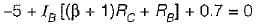or,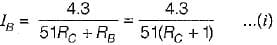Now,.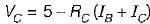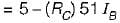or,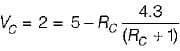(Using equation (i))
or, RC = 2.3 kΩ

Test: Transistor Biasing & Thermal Stabilization- 2 - Question 4

Assuming the current source to be ideal, all transistors to be identical, and IC = βIB the stability factor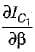for the current mirror circuit shown below would be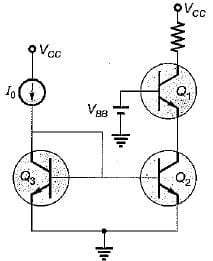Detailed Solution for Test: Transistor Biasing & Thermal Stabilization- 2 - Question 4

Applying KCL at the collector of Q3 gives,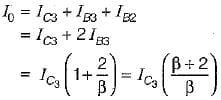or,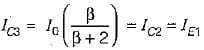Also,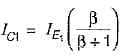Hence,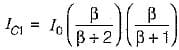Therefore,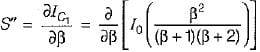or,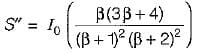Test: Transistor Biasing & Thermal Stabilization- 2 - Question 5

A reverse biased diode placed in parallel with the base-emitter junction makes the circuit insensitive to variations in______with changes in temperature.

Detailed Solution for Test: Transistor Biasing & Thermal Stabilization- 2 - Question 5

The circuit for the “diode compensation for I"CO is shown below.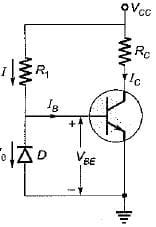Here, if the diode and the transistor are of the same type and material, the reverse saturation current I0 of the diode will increase with temperature at the same rate as the transistor current ICO.
Here,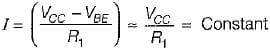Since the diode is reverse biased by VBE = 0.2 V (for Ge device), the current through D is I0 and IB = I - I0.
Now,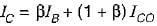Thus, if β ≫ 1 and if I0 of D and ICO of transistor track each other over the desired temperature range, then IC remains essentially constant so that the circuit becomes insensitive toe variations in ICO with changes in temperature.

Test: Transistor Biasing & Thermal Stabilization- 2 - Question 6

The static (DC) and dynamic (AC) load lines in a transistor amplifier are different when

Test: Transistor Biasing & Thermal Stabilization- 2 - Question 7

Thermal runaway is not possible in FET because as the temperature of FET increases,

Test: Transistor Biasing & Thermal Stabilization- 2 - Question 8

The two transistors in figure are identical. If β = 25, the current IC2 is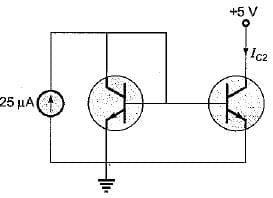Detailed Solution for Test: Transistor Biasing & Thermal Stabilization- 2 - Question 8

Both transistor are in the forward active region.
Here, IC1 + IB1 + IB2 = 25 μA
Since the transistors are identical and have the same VBE, therefore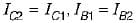∴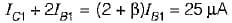or,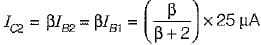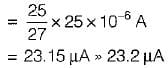Test: Transistor Biasing & Thermal Stabilization- 2 - Question 9

The input resistance of a common emitter stage can be increased by
1. unbypassing emitter resistance
2. bootstrapping
3. biasing it at low quiescent current
4. using compounded BJTs

Q. The correct sequence in descending order of the effectiveness of these methods is

Test: Transistor Biasing & Thermal Stabilization- 2 - Question 10

For the transistor amplifier shown in figure below, VCC = 12 V, R1, = 8 kΩ, R2 = 4 kΩ, RC = 1 kΩ, RE = 1 kΩ.
Assuming VBE = 0.7 V, the operating point Q be given as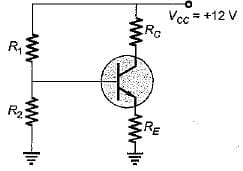Detailed Solution for Test: Transistor Biasing & Thermal Stabilization- 2 - Question 10

The voltage across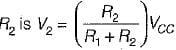Therefore,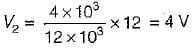Now,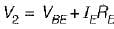∴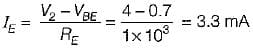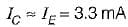∴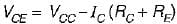= 12 - 3.3 x 10-3 x 2 x 103
= 5.4 volt
∴ Q = (5.4 V, 3.3 mA)

Test: Transistor Biasing & Thermal Stabilization- 2 - Question 11

In a self bias circuit using BJT, if the stability factor is represented as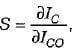then the rate of change of IC w.r.t. β, keeping ICO and VBE constant will be given by (IC= collector current of the transistor)

Detailed Solution for Test: Transistor Biasing & Thermal Stabilization- 2 - Question 11

Stability factor S is given by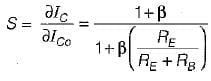Now,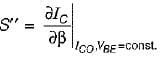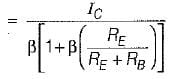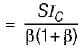Test: Transistor Biasing & Thermal Stabilization- 2 - Question 12

The forward current gain (α) of a bipolar transistor can be increased by
1. reducing the recombination lifetime in the emitter
2. increasing the emitter doping density.
3. increasing the base doping density.
4. reducing the base width.
Select the correct code from the given options,

Detailed Solution for Test: Transistor Biasing & Thermal Stabilization- 2 - Question 12

• When emitter is heavily doped, more majority carriers will reach into base due to which lC will increase and hence α increases.
• Due to early effect, base width is reduced as a result of which IC is slightly increased. Hence, α of the transistor is increased.
Hence, 2 and 4 are correct.

Test: Transistor Biasing & Thermal Stabilization- 2 - Question 13

What is the value of thermal resistance for the 2N338 transistor for which the manufacturer specifies PC,max = 125 mW at 25°C free-air temperature and maximum junction temperature of 150°C?

Detailed Solution for Test: Transistor Biasing & Thermal Stabilization- 2 - Question 13

Given,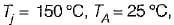PC,max = 125 mW
We know that,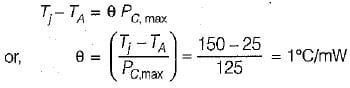Test: Transistor Biasing & Thermal Stabilization- 2 - Question 14

The stability factor (S1) for the circuit shown below is given by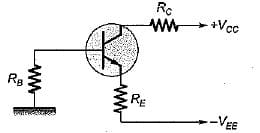Detailed Solution for Test: Transistor Biasing & Thermal Stabilization- 2 - Question 14

Applying KVL, we get: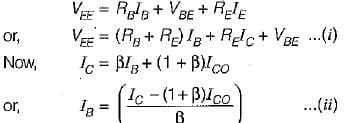Putting value of lB from equation (ii) into (i), we get: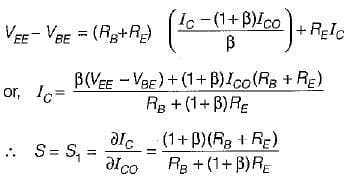Test: Transistor Biasing & Thermal Stabilization- 2 - Question 15

For the cascade amplifier shown below, the quiescent current through transistors is 1.mA and the collector voltage VC2 = 6 V and VC1 = 3 V. Assuming VBE = 0.7 V and very high Value of β, the values of R1 and R2 will be respectively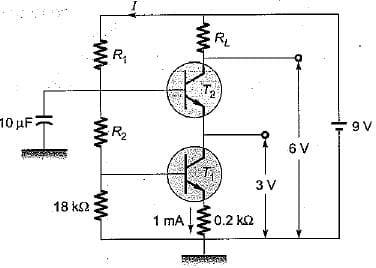Detailed Solution for Test: Transistor Biasing & Thermal Stabilization- 2 - Question 15

Since β is very large, we can neglect the base current of the transistors.
Now,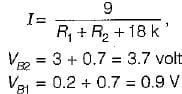Hence, current through 18 kΩ resistor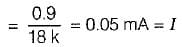∴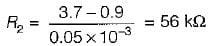and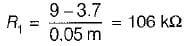## Topicwise Question Bank for Electrical Engineering

207 tests
 Use Code STAYHOME200 and get INR 200 additional OFF Use Coupon Code
Information about Test: Transistor Biasing & Thermal Stabilization- 2 Page
In this test you can find the Exam questions for Test: Transistor Biasing & Thermal Stabilization- 2 solved & explained in the simplest way possible. Besides giving Questions and answers for Test: Transistor Biasing & Thermal Stabilization- 2, EduRev gives you an ample number of Online tests for practice

207 tests